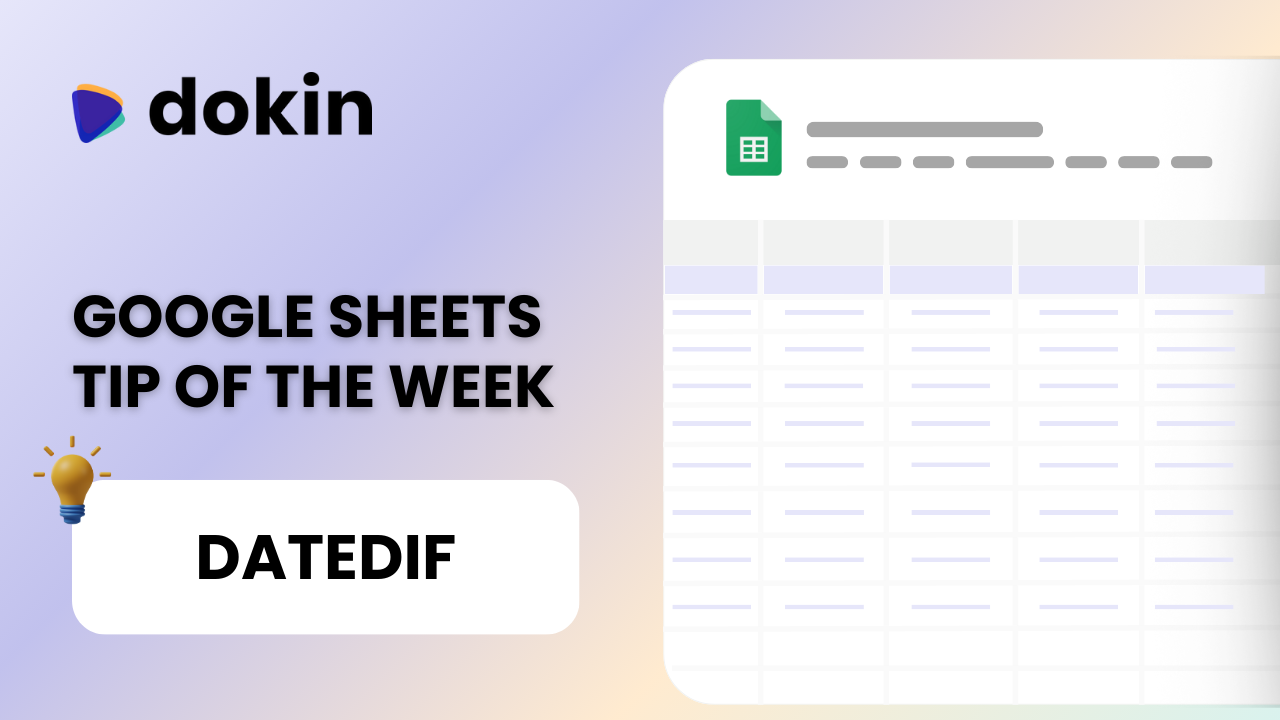/
November 5, 2023## Introduction

If you've ever needed to calculate the difference between two dates in Google Sheets, you'll understand the potential challenges that come with it. While basic subtraction can give you the difference in days, what if you are looking for results in months or years? Here's where the DATEDIF function steps in as a versatile tool. In this article, we'll dive into how to harness the power of the DATEDIF function in Google Sheets to calculate date differences and address some common queries.### Did you know?

Dokin allows you to sync live data from your company systems with Google Sheets, Slides and Docs.

Feed live data to your dashboards and automate reporting.

## Understanding DATEDIF in Google Sheets

### How Do I Use DATEDIF in Google Sheets?

The DATEDIF function is a versatile tool that simplifies date calculations in Google Sheets. You can use it to determine the difference between two dates in terms of days, months, or years. There are many use cases that may require you to calculate the difference between two dates. For example, you may need to calculate the age of a certain receivable or debt, the seniority of an employee etc. DATEDIF comes in handy in such cases.

Let's break it down into simple steps:

• In an empty cell, type the DATEDIF function using the following syntax: =DATEDIF(start_date, end_date, unit).
• For the start_date, specify the date from which you want to calculate the difference. This can be a cell reference, a function returning a date, or a date value.
• For the end_date, indicate the date up to which you want to calculate the difference.
• Finally, select the unit parameter, specifying whether you want the result in "days" ("D"), "months" ("M"), or "years" ("Y"). Accepted values are "Y","M","D" ,"MD","YM","YD".

• "Y": the number of whole years between start_date and end_date.

• "M": the number of whole months between start_date and end_date.

• "D": the number of days between start_date and end_date.

• "MD": the number of days between start_date and end_date after subtracting whole months.

• "YM": the number of whole months between start_date and end_date after subtracting whole years.

• "YD": the number of days between start_date and end_date, assuming start_date and end_date were no more than one year apart.

Now, you can calculate date differences with ease, without manual computations.

### How to Calculate Dates in Google Sheets - Example

The DATEDIF function empowers you to handle a wide range of date-related calculations in Google Sheets. It's a valuable tool for quickly determining the time intervals between dates, and it can save you from the hassle of manual calculations.

In the example below, we want to calculate the seniority of each employee, in months.

To do so, we are going to use DATEDIF. As seen before, the formula should be:
=DATEDIF(start_date, end_date, unit).

Therefore in F9 we are going to enter =DATEDIF(E9,\$C\$6,"M")where M returns the difference in months.

If instead we wanted the difference in days, we’ll substitute the unit character from “M” to “D”:

As I mention in my Youtube tutorial, one point to keep in mind is that DATEDIF returns the difference in whole numbers. Months and years are only counted if they are equal to or go past the "day." For example, the function returns "4 months" between the dates 9/30/15 and 2/28/16 (even though the 28th is the last day of the month).

### Datedif in Google Sheets Years and Months

If you need to calculate differences in years and months between two dates, the DATEDIF function is your go-to solution. By using "YM," "YD," and "MD" as the unit parameter, you can easily obtain remainders in months or days after dividing by years or months.

## Conclusion

When it comes to finding the difference between two dates in Google Sheets, the DATEDIF function offers flexibility beyond simple subtraction. You can choose the units in which you want the difference returned: days, months, or years.

Now, you're equipped with the knowledge and tools to handle date calculations in Google Sheets with confidence and efficiency. Embrace the power of DATEDIF and the seamless data integration offered by Dokin to streamline your data management and reporting.